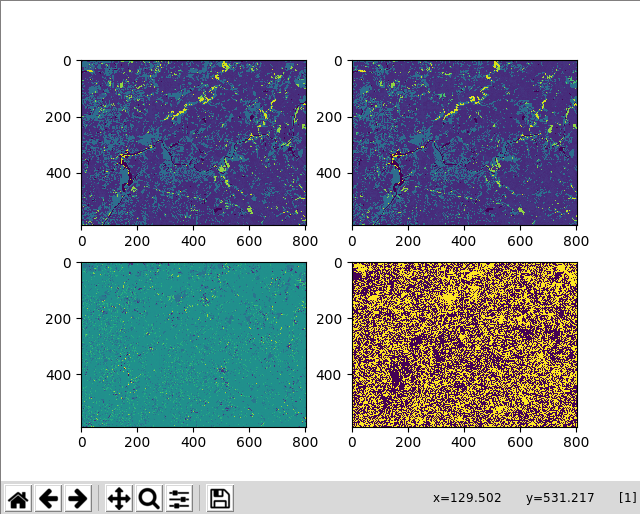# How to detect changes between two GeoTIFF files using NumPy

Institute for Environmental and Spatial Analysis...University of North Georgia

## 1   Introduction

Try these exercises first:

In this exercise, we’ll try to detect changes between two GeoTIFF files. Use nlcd2001_clipped.tif and nlcd2016_clipped.tif. For `np.where()`, see this exercise.## 2   Python

``````from osgeo import gdal
import numpy as np
import matplotlib.pyplot as plt

ds = gdal.Open(filename)
band = ds.GetRasterBand(1)
del ds, band
return arr

nlcd_changes = nlcd16_arr - nlcd01_arr

nlcd_changed = np.where(nlcd_changes != 0, 1, 0)

plt.subplot(221)
plt.imshow(nlcd01_arr)

plt.subplot(222)
plt.imshow(nlcd16_arr)

plt.subplot(223)
plt.imshow(nlcd_changes)

plt.subplot(224)
plt.imshow(nlcd_changed)

plt.show()``````

## 3   Explanation

Import necessary modules and define the read_geotiff() function.

``````from osgeo import gdal
import numpy as np
import matplotlib.pyplot as plt

ds = gdal.Open(filename)
band = ds.GetRasterBand(1)
del ds, band
return arr``````

Read data as 2-dimensional NumPy arrays.

``````nlcd01_arr = read_geotiff("nlcd2001_clipped.tif")

NumPy supports very simple algebraic operations between arrays. In this case, we want to subtract the old 2001 NLCD class values from the new 2016 ones. If there were no changes between the two years i some locations, the resulting cell values at those no-change locations would be 0 because we subtract class values from class values. If there were changes, those cells would be assigned positive or negative values.

``nlcd_changes = nlcd16_arr - nlcd01_arr``

Let’s create a binary array that contains 1 for changed cells and 0 for the rest. We use `np.where()` and the `nlcd_changes != 0` condition. What this expression means is that if `nlcd_changes` is not 0 (NLCD changed), use 1. Otherwise (NLCD didn’t change), use 0.

``nlcd_changed = np.where(nlcd_changes != 0, 1, 0)``

Since `nlcd01_arr`, `ncd16_arr`, `nlcd_changes`, and `nlcd_changed` are all 2-dimensional arrays, we can use `plt.imshow()` to plot them.

``````plt.subplot(221)
plt.imshow(nlcd01_arr)

plt.subplot(222)
plt.imshow(nlcd16_arr)

plt.subplot(223)
plt.imshow(nlcd_changes)

plt.subplot(224)
plt.imshow(nlcd_changed)

plt.show()``````